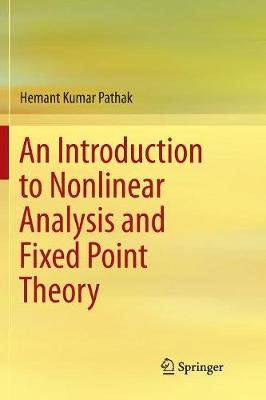•# An Introduction to Nonlinear Analysis and Fixed Point Theory (Paperback)

(author)
£69.99
Paperback 830 Pages / Published: 23/12/2018
• We can order this

Usually dispatched within 3 weeks

This book systematically introduces the theory of nonlinear analysis, providing an overview of topics such as geometry of Banach spaces, differential calculus in Banach spaces, monotone operators, and fixed point theorems. It also discusses degree theory, nonlinear matrix equations, control theory, differential and integral equations, and inclusions. The book presents surjectivity theorems, variational inequalities, stochastic game theory and mathematical biology, along with a large number of applications of these theories in various other disciplines. Nonlinear analysis is characterised by its applications in numerous interdisciplinary fields, ranging from engineering to space science, hydromechanics to astrophysics, chemistry to biology, theoretical mechanics to biomechanics and economics to stochastic game theory. Organised into ten chapters, the book shows the elegance of the subject and its deep-rooted concepts and techniques, which provide the tools for developing more realistic and accurate models for a variety of phenomena encountered in diverse applied fields. It is intended for graduate and undergraduate students of mathematics and engineering who are familiar with discrete mathematical structures, differential and integral equations, operator theory, measure theory, Banach and Hilbert spaces, locally convex topological vector spaces, and linear functional analysis.

Publisher: Springer Verlag, Singapore
ISBN: 9789811342615
Number of pages: 830
Weight: 1294 g
Dimensions: 235 x 155 mm
Edition: Softcover reprint of the original 1st ed. 201